# Quiz 3: Arcticl Insulation

Business

Economic rate of return: The economic rate of return is defined as the discount rate in any investment, which equated the present value of its expected net revenue to the initial outlay. It is considered as an important parameter while planning strategies related to investment. Calculate the economic return for the period: Methodology: Economic rate of return is defined as the discount rates, which makes the net present value zero.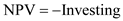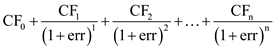Where, The net present value as NPV The annual cash flows as CF The production year as n The economic rate of return as err The economic rate of return is trial and error method. The criterion of making decision is as follows: • Exclusive cases: To select the investment plan, which has the biggest, ERR results among the various investment plans. • Independent cases: To select the investment plan, which has, ERR results over WACC within the budget. This implies that if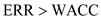, the project is to be accepted. The economic return for the period: The cash flow forecast: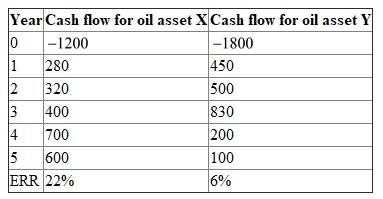The assumptions made are: X-oil asset: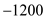investment Y-oil asset: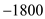investment WACC: 15% X-Oil investment, the project is accepted as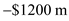Y-Oil investment, the project is rejected as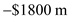Internal accounting method: When decision-making depends on the internal financial statements internal accounting method is adopted which is aligned with factors like valuation purpose, obtaining finance, day-to-day operations. Best internal accounting method for fuel gas and residual oil: • Full cost method is considered as the best internal accounting method for fuel gas and residual oil. • All property acquirement, investigation and improvement costs, even dry hole costs, are capitalized as the properties of oil and gas. • Unit-of-production method is used for amortization of the above costs which is based on volumes delivered and the reserves. • It allows all the operating expenses relating to exploration of new oil and natural gas reserves-regardless of any outcomes to be capitalized. Reason as to why the full cost method is considered best: • This method is considered because it views the realities of exploring and developing oil and gas reserves. • Therefore, all the costs are first capitalized and then written off over the course of full operating cycle.

Economic return: Economic return is defined as the interest rate where the cost of the project and benefit of the project become equal when they are discounted over its life. Accounting numbers producing economic returns: Economic rate of return is the rate of return on the amount which is invested in an economic entity in order to decide whether or not to undertake an investment. The accounting numbers used for calculating economic returns are: • Gain from the investment: Gain form investment is defined as the income, which is obtained from selling an investment for an amount, which is greater than its purchase price. • Cost of investment: Cost of investment is defined as the cost, which is needed beyond the development phase to introduce new capability into operational use, to procure additional, initial or replacement for equipment's for operational use. • Net present value: Net present value is defined as the difference between the present value of the cash outflows and the present value of cash inflows. • Internal rate of return: Internal rate of return is defined as the rate of return, which is used in the capital budgeting technique for measuring and comparing the profitability of investments. • Accounting rate of return: Accounting rate of return is defined as the ratio of projected accounting revenue from the project to the average investment made in the project.